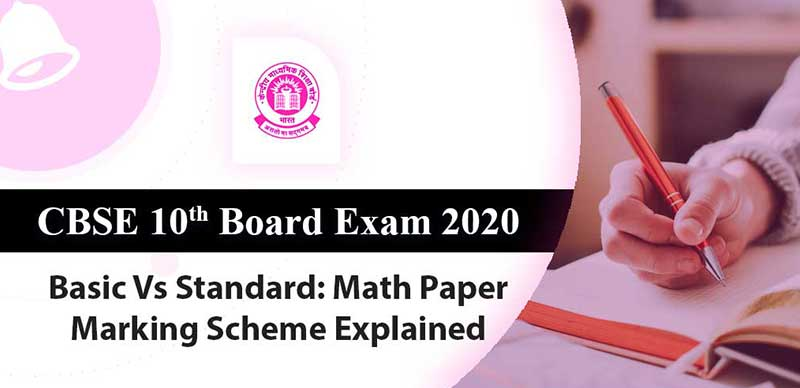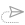### CBSE 10th Board Exam 2020: Basic Vs Standard - Math Paper Marking Scheme Explained

CBSE has introduced two levels of Mathematics in class 10 from session 2019-20. In March 2020 there will be two different levels of examinations for class 10 Mathematics.

These are Mathematics-Basic and Mathematics-Standard. However, the syllabus and textbooks for both the levels are same but the question papers will be different. The question paper of Mathematics-Basic will be easy whereas Mathematics-Standard will be same as earlier.

The Mathematics exam is scheduled on March 12. Exam for both levels will be held on the same day.

What is the difference between the two papers? In simple terms, Basic Mathematics is supposed to be easier than Standard Mathematics. But that is not the only case.

The difference in the two papers is not limited to the difficulty-level of the paper. As the marking scheme and curriculum explain, the weightage allocated to different components of the curriculum also varies between Basic and Standard Mathematics.

In case of Basic Mathematics, 32 marks are allotted to questions which require remembering facts, terms, concepts and answers. In case of Standard mathematics, 20 marks' worth of questions will require remembering facts, terms and concepts.

Similar difference is present for other components. In Basic Mathematics paper, 28 marks are allotted for questions which would demonstrate a student's understanding of concepts. In Standard mathematics paper, it is worth 23 marks.

Application based questions will carry 12 marks in basic Mathematics paper and 19 marks in Standard Mathematics paper. and last but not the least, Questions which require analyzing, evaluation and compiling of information will carry 8 marks in Basic Maths paper and 18 marks in Standard Maths paper.

#### Terminologies:

Mathematics – Standard: For the existing level of examination.

Mathematics – Basic: For the easier level of examination

The Standard level will be meant for students who wish to opt for Mathematics at Senior Secondary level and the Basic level would be for students not keen to pursue Mathematics at higher levels.

#### No difference in the syllabus:

As per the official circular, “the syllabus, classroom teaching and internal assessment for both the levels of examination would remain the same; so that the students get an opportunity to study the whole range of topics throughout the year and are able to decide upon the level of Board examination depending upon their aptitude and abilities.”

#### Not applicable to the internal assessment in CBSE Class 10

The two levels of Examination will be held in the subject of Mathematics in CBSE Board Exams 2020 of Class 10 and the same shall not be applicable to the internal assessment in class 10.

#### Not applicable for CBSE Class 9

There shall not be two levels of Assessment/Examination for class IX.

#### Procedure to opt for the paper

A student will have the right to choose between the two levels of Examination at the time of submission of List of Candidates (LoC) by the affiliated school to the Board online.

#### What if a student fails in the exam?

In case student fails at any level of Mathematics, he/she can appear at the compartment examination as per norms of the Board according to the options given below:

 Failed in Compartment options Mathematics -Basic Mathematics-Basic Mathematics-Standard Mathematics Standard or Mathematics- Basic

#### To Summarise

Choose Maths Basic if you want to

• Take arts in Class 11 and 12
• Take Commerce without Maths in Class 11 and 12
• Take any non-maths course after Class 12 - like Design, Animation, Humanities

Choose Maths Standard if you want to

• Take Commerce with Maths in Class 11 and 12
• Take Science in Class 11 and 12
• Give IIT-JEE or Medical Exams (NEET)
• To do any Engineering course after Class 12
• To do CA, CS after Class 12
• Want to go to Medical Field after Class 12

Question: What is the difference between the Standard Level and Basic Level of Mathematics in CBSE Class 10 Board Exams 2020?

The existing Mathematics examination is the Standard Level Examination.

Standard-Level and Basic-level Question papers shall be based on the same syllabus. However, the Standard-Level Mathematics assesses higher Mathematical abilities compared to Basic-Level. Accordingly, the difficulty level of the Mathematics – ‘Basic’ is less than that of Mathematics-‘Standard’.

Question: Is CBSE increasing the burden on the child by introducing the concept of two Levels of Exams in Mathematics?

No, because the option to pick either Level is with the student. Further, the syllabus prescribed by CBSE will remain the same for both the levels.

Question: Will this lead to dilution of Mathematics syllabus/teaching?

No, the idea is to make the evaluation more students centric and reduce the pressure on the student who doesn’t want to pursue Mathematics after class 10.

Question: Can a student opt for both Standard Level and Basic Level Exams?

No. There is only one option, either Mathematics-Standard or Mathematics-Basic.

Question: To continue Mathematics as a subject in class 11/12 and further, which Level Exam does a student need to pass?

Mathematics - Standard is to be passed at class X, for taking up Mathematics at Senior Secondary level.

Question: If a student qualifies only the Mathematics-Basics in class X, can he/she opt for Mathematics in class 11/12?

No. If he/she intends to take Mathematics in class 11/12, he/she has to pass Mathematics-Standard.

Question: If a student has registered for the Basic Level Exam but wishes to appear for the Standard Level after declaration of result, can he/she do so?

Yes. One can appear in the Standard Level at the Compartment examination, if one wishes to do so after passing the Basic Mathematics Examination.

Question: How I can get the CBSE Sample Papers and question paper designs of Standard Level and Basics level Mathematics papers?

CBSE Sample papers and Blue Print based on the curriculum for 2019-20 will be made available on: www.cbseacademic.nic.in. in due course of time.##### WBJEE Answer Key 2020: Released

17 February, 2020, 1:18 pm##### CBSE Board Exam 2020: Check list of barred items, which pen to use, timings & other details

15 February, 2020, 12:30 pm##### CBSE Board Exam 2020: Board asks students to follow these rules

14 February, 2020, 12:35 pm##### CBSE Board Exam 2020: Importance of word limit in exam writing

13 February, 2020, 1:18 pm##### CBSE 10th Board Exam 2020: Basic Vs Standard - Math Paper Marking Scheme Explained

12 February, 2020, 1:40 pm##### CBSE Board Exam 2020: How to utilise additional 15 minute reading time in exams?

11 February, 2020, 1:52 pm